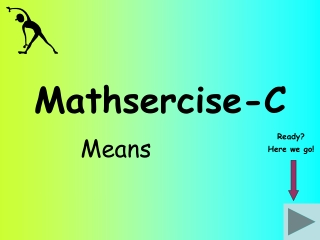DownloadDownload PresentationMathsercise-C

# Mathsercise-C

Télécharger la présentation## Mathsercise-C

- - - - - - - - - - - - - - - - - - - - - - - - - - - E N D - - - - - - - - - - - - - - - - - - - - - - - - - - -
##### Presentation Transcript

1. Mathsercise-C Means Ready? Here we go!

2. First you need two extra columns! Frequency No. of hours 0 < h ≤ 20 8 7 20 < h ≤ 40 5 40 < h ≤ 60 The table shows how much TV 20 students watched in a week Midpoint FxM Question 2 10 80 210 30 50 250 20 540 Work out an estimate for the mean number of hours that students watched TV To find the estimate of the mean, add the FxM up and divide by the 20 students ÷ Answer The midpoint is half way through each class interval = 27 hours 1 Means

3. Frequency Amount (£) 0 < t ≤ 4 11 3 4 < h ≤ 8 11 8 < h ≤ 12 This table shows how much money 25 students had at school Midpoint FxM Question 3 2 22 6 18 10 110 25 150 Work out an estimate for the mean amount of money that each student has Answer = £6 ÷ 2 Means

4. Day Mon Tue Thu Fri Sat Wed No. 12 8 13 15 14 19 The table shows the number of flower bouquets delivered each day of the week Question 4 12 8 13 12 14 16 Work out the 3-day moving average for this data Answer 3-day moving average means the average of each set of 3 days + + = 33 ÷ 3 = 11 8 + 13 + 15 = 36 ÷ 3 = 12 3 Means

5. The table shows the daily takings for an ice-cream salesman Day Mon Tue Thu Fri Sat Sun Wed (£) 50 36 24 90 130 156 264 Start 50 70 100 160 Notice where these boxes are positioned! Work out the 4-day moving average for this data This time find the average of 4 values at a time 4 Means Answer